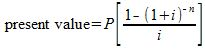# How to Calculate the Present Value of an Annuity

Instructions:

Choose an answer and hit 'next'. You will receive your score and answers at the end.

question 1 of 3

### What does the P stand for in this formula?Create Your Account To Take This Quiz

As a member, you'll also get unlimited access to over 75,000 lessons in math, English, science, history, and more. Plus, get practice tests, quizzes, and personalized coaching to help you succeed.

Try it risk-free for 30 days. Cancel anytime.

### 2. Calculate the present value of annuity with fixed payments of \$500, annual interest rate of 4%, and a total of 3 annual payments.

Create your account to access this entire worksheet
A Premium account gives you access to all lesson, practice exams, quizzes & worksheets
Quizzes, practice exams & worksheets
Certificate of Completion
Create an account to get started

The quiz will test you on the formulas and definitions related to present value. Some other questions will ask you to calculate the present value of an annuity.

## Quiz & Worksheet Goals

The quiz will test you on the following:

• Present value
• Annuity
• Present value formula
• Interest rates

## Skills Practiced

You will practice these following skills:

• Reading comprehension - ensure that you draw the most important information from the related present value of an annuity lesson
• Problem solving - use acquired knowledge to solve present value practice problems
• Interpreting information - verify that you can read information regarding interest rates
• Information recall - access the knowledge you've gained concerning the present value of an annuity

Finish the quiz and head over to the corresponding lesson How to Calculate the Present Value of an Annuity. The lesson covers the following topics:

• Exploring the definition of annuity
• Relating annuity to present value
• Explaining fixed payment amounts
• Calculating present values of annuities
Final Exam